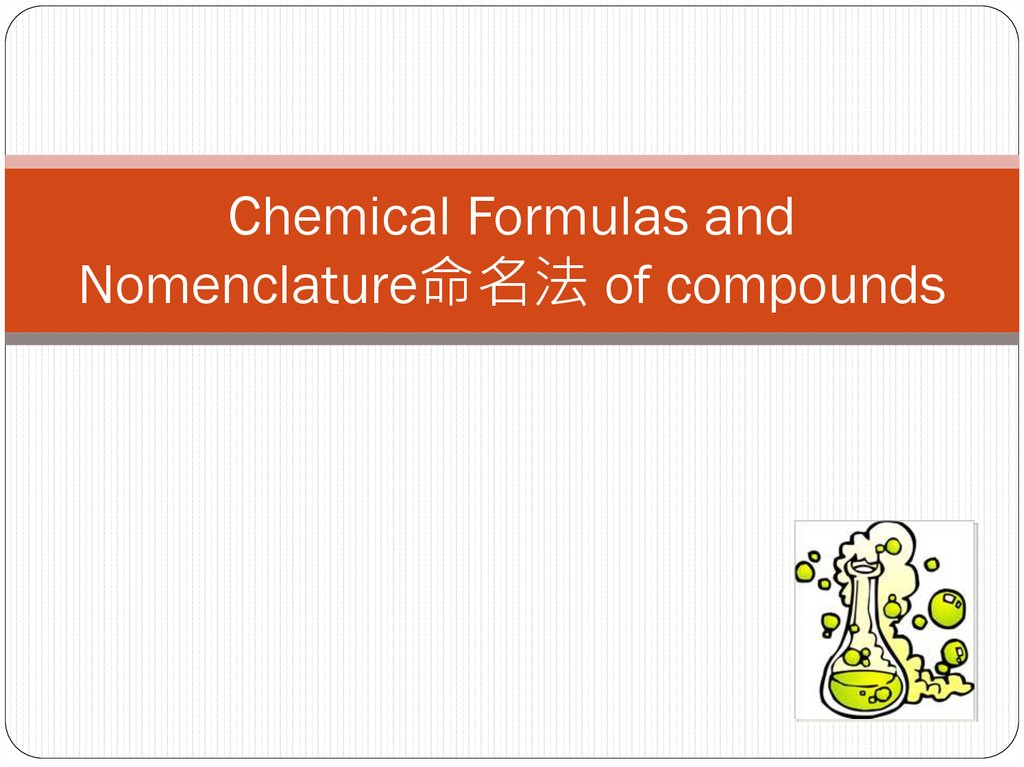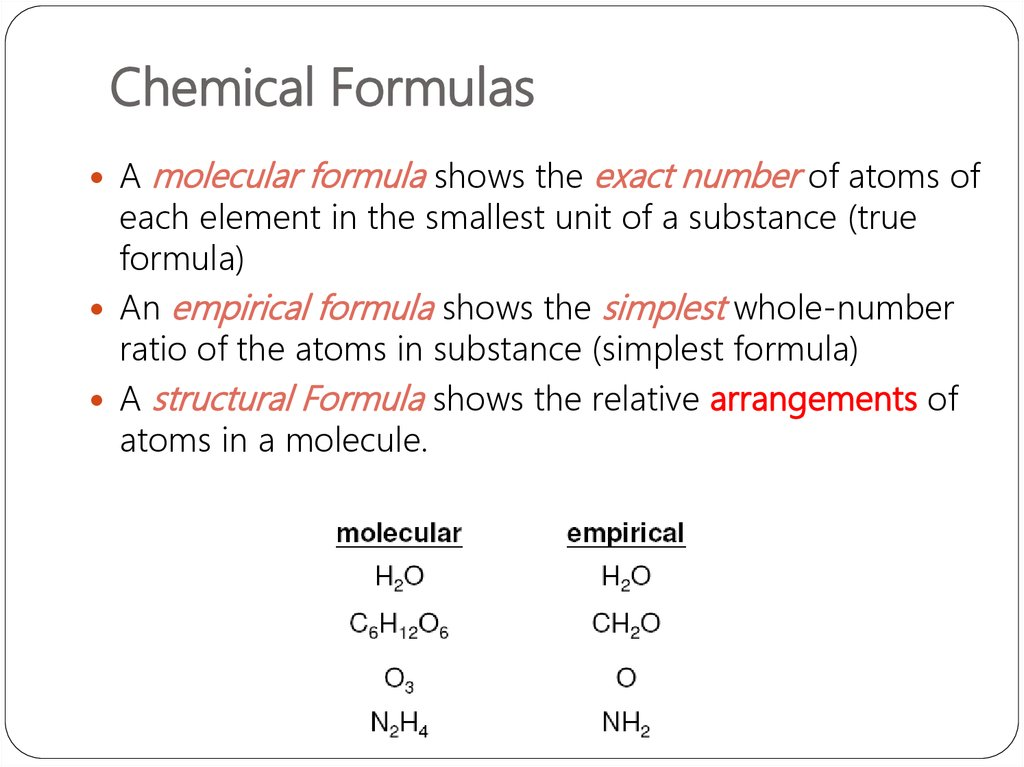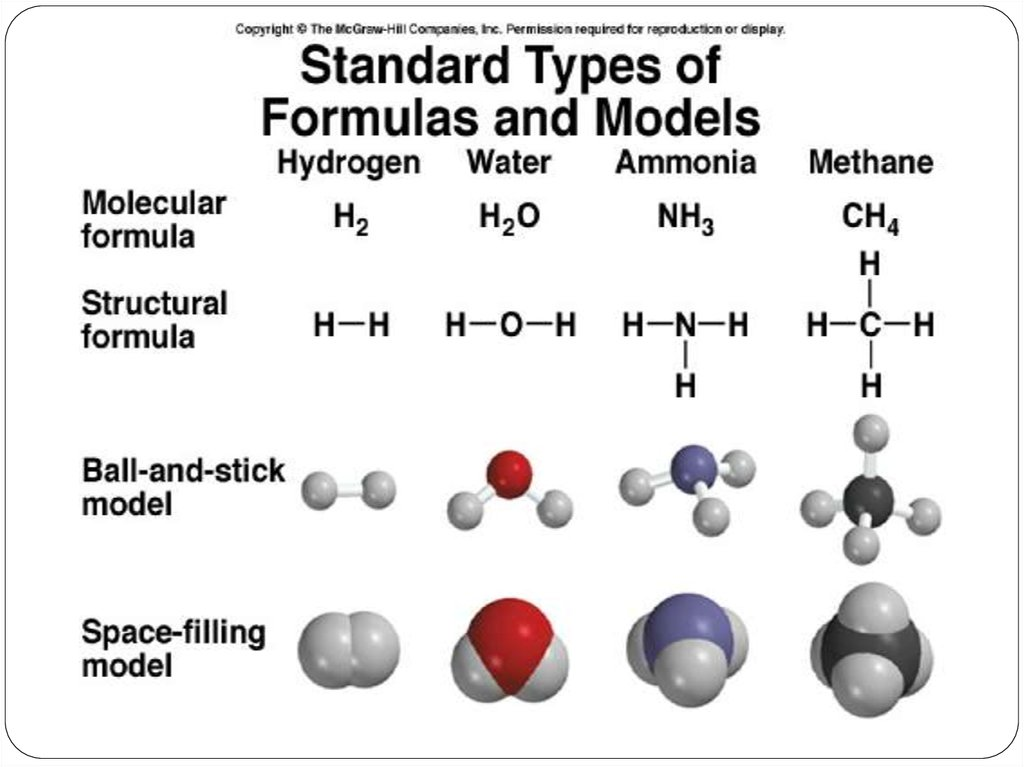# Chemical Formulas and Nomenclature of compounds

## 2. Chemical Formulas

A
molecular formula shows the exact number of atoms of
each element in the smallest unit of a substance (true
formula)
An empirical formula shows the simplest whole-number
ratio of the atoms in substance (simplest formula)
A structural Formula shows the relative arrangements of
atoms in a molecule.

## 4.

If you know the name of an ingredient, you can write a
chemical formula, and the percent composition of a particular
substance can be calculated from the formula. This can be
useful information for consumer decisions.

## 14.

Ionic compounds consist of a combination of cations and
anions (metal + nonmetal).
• the formula is always the same as the empirical formula.
• the sum of the charges on the cation(s) and anion(s) in each
formula unit must equal zero.
The ionic compound NaCl

## 17.

Formula of Ionic Compounds
2 x +3 = +6
Al3+
1 x +2 = +2
Ca2+
3 x -2 = -6
Al2O3
O22 x -1 = -2
CaBr2
Br-
1 x +2 = +2
1 x -2 = -2
Na2CO3
Na+
CO32-

## 22. Chemical Nomenclature

Ionic Compounds
often a metal + nonmetal
anion (nonmetal), add “ide” to element name
BaCl2
barium chloride
K2O
potassium oxide
Mg(OH)2
magnesium hydroxide

## 23.

Transition metal ionic compounds
indicate charge on metal with Roman numerals羅馬數字
FeCl2
2 Cl- -2, so Fe is +2
iron(II) chloride
FeCl3
3 Cl- -3, so Fe is +3
iron(III) chloride
Cr2S3
3 S-2 -6, so Cr is +3 (6/2)
chromium(III) sulfide
iron(II) chloride
iron (II) chloride

## 31.

Molecular compounds
• nonmetals or nonmetals + metalloids
• common names
H2O, NH3, CH4, C60
• element further left in periodic table is
1st
• element closest to bottom of group is
1st
• if more than one compound can be
formed from the same elements, use
prefixes to indicate number of each
kind of atom
• last element ends in ide

## 32.

Molecular Compounds
HI
hydrogen iodide
NF3
nitrogen trifluoride
SO2
sulfur dioxide
N2Cl4
NO2
N2O
TOXIC!
Laughing Gas